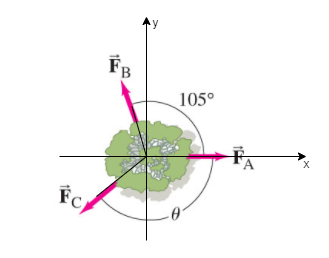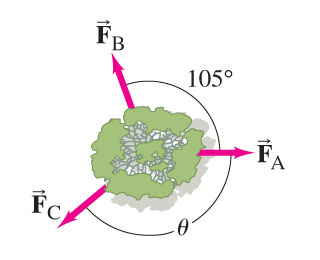# Problem: Three forces are applied to a tree sapling, as shown in (Figure 1) , to stabilize it. Suppose that FA→ = 355 Nand FB→ = 475 N .Part ADetermine the magnitude of FC→ .Express your answer to three significant figures and include the appropriate units.Part BDetermine the angle between FA→  and FC→ measured clockwise. Express your answer using three significant figures.

###### FREE Expert Solution

Let's label the axes and have teh diagram as,Part A

From our diagram,

FBy = FBcos(105° - 90°) = (475)cos(15°) = 458.1 N

FBx = FBsin(105° - 90°) = (475)sin(15°) = 122.94 N

Therefore, the forces along the x-direction are:

87% (57 ratings)###### Problem Details

Three forces are applied to a tree sapling, as shown in (Figure 1) , to stabilize it. Suppose that $\stackrel{\to }{{\mathrm{F}}_{\mathrm{A}}}$ = 355 Nand $\stackrel{\to }{{\mathrm{F}}_{\mathrm{B}}}$ = 475 N .Part A

Determine the magnitude of $\stackrel{\to }{{\mathrm{F}}_{\mathrm{C}}}$ .

Express your answer to three significant figures and include the appropriate units.

Part B

Determine the angle between $\stackrel{\to }{{\mathrm{F}}_{\mathrm{A}}}$  and $\stackrel{\to }{{\mathrm{F}}_{\mathrm{C}}}$ measured clockwise. Express your answer using three significant figures.

Frequently Asked Questions

What scientific concept do you need to know in order to solve this problem?

Our tutors have indicated that to solve this problem you will need to apply the Vector Composition & Decomposition concept. You can view video lessons to learn Vector Composition & Decomposition. Or if you need more Vector Composition & Decomposition practice, you can also practice Vector Composition & Decomposition practice problems.

What professor is this problem relevant for?

Based on our data, we think this problem is relevant for Professor Jerousek's class at UCF.